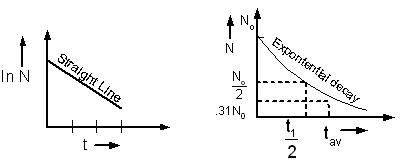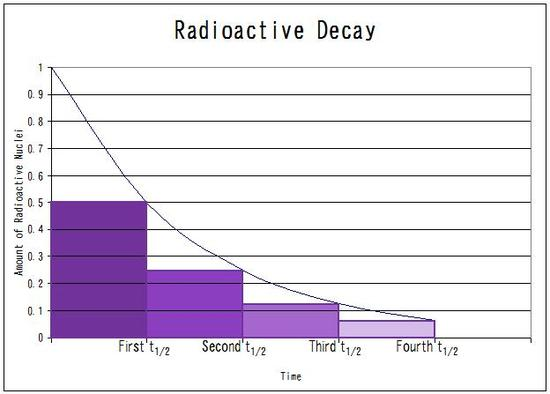×#### Thank you for registering.

One of our academic counsellors will contact you within 1 working day.

Click to Chat

1800-1023-196

+91-120-4616500

CART 0

• 0

MY CART (5)

Use Coupon: CART20 and get 20% off on all online Study Material

ITEM
DETAILS
MRP
DISCOUNT
FINAL PRICE
Total Price: Rs.

There are no items in this cart.
Continue Shopping• Complete Physics Course - Class 11
• OFFERED PRICE: Rs. 2,968
• View Details

```Radioactive Decay Law

Table of Content

Related Resources

Radioactivity is a vital component of Modern Physics. The IIT JEE often picks up questions form this topic and hence, it becomes very essential for the JEE aspirants to master this topic in order to remain competitive in the JEE. Questions may sometimes be asked on the derivation of radioactive decay constant or the derivation of radioactive rate law.

The radioactive decay law explains or clarifies how the number of non-decayed nuclei of a given radioactive substance falls in due course of time.

The number of atoms disintegrating per second γ is very small in the SI system it take a large number N (~ Avogadro number, 1023) to get any significant activity.

dN /dt = – γN               ….(i)

Where γ is the radioactivity decay constant.

If N0 is the number of radioactive atoms present at a time t = 0, and N is the number at the end of time t, then

N = N0e– γt

The term (–dN/dt) is called the activity of a radioactive substance and is denoted by 'A'.

The decay constant may be characterized in two ways: mean life and half-life. The unit of measurement in both the cases is the same i.e. seconds. Mean-life as the name suggests is the average of the lifetime of an element. It may be represented by the following expressions:

Nt = Noe−λt

Rearranging the equation we get,

C = λ/No

Now we discuss the concept of half-life. Half-life describes the time period required by half of the substance to decay. The rate of decay remains constant throughout the decay process. The exponential nature of half-life may be represented in either of the following ways:

Nt =No (1/2) t/t1/2

Nt = Noe−t/τ

By comparing these equations, we get following expressions

ln (1/2)t/ t1/2 = ln (e-t/τ) = ln (e -λt)

or with ln(e) = 1, thenBy cancelling t on both sides, the following equation of half-life is obtained

t1/2 = d frac ln (2) λ= 0.693λ

Clearly, the above equation is constant meaning that the half-life of a radioactive substance is constant.

1 Becquerel (Bq) = 1 disintegration per second (dps)

1 curie (Ci)          = 3.7 x 1010 dps

1 Rutherford        = 106 dps

The changes in N, due to radioactivity, are very small in 1 s compared with the value of N itself.

Therefore, N may be approximated by means of a continuous function N (t).

For the case considered in equation (i), we can write,

A = rate of decrease of N = λN

or –dN/dt = λN                                       ..... (ii)

Note the equation (ii) is valid only for large value of N, typically –1018 or so.

If the number of atoms at time t = 0 (initial) is N = N0, then one can solve equation (ii):or     ln N/N0 = –λt

or     N(t) = N0 e–λt                                        ......(iii)

The activity A (t) = λN (t) and is given by

A (t) = λN0

= A0 λN0)           .... (iv)

The variation of N is represented below:The graph given below shows the radioactive decay during the various half-lives. Radioactive decay is not always a single step phenomenon. Mostly, the parent nuclei get transformed into daughter nuclei which are also radioactive and hence decay. It is quite possible that in such cases the half-life of the parent nuclei may be longer or shorter than the half-life of the daughter nuclei. Depending on the type of substance, there might arise a case when the half-life of both parent and daughter nuclei are same.Watch this Video for more reference

Radioactive decay law lays the groundwork for all the topics of radioactivity. It is an interesting topic and must be given due importance. askIITians brings you the study material containing several solved examples on the radioactive law. It is important to gain proficiency in this area to fare well in JEE.

Related Resources

Look into the Solved Papers of Past Years to get a hint of the kinds of questions asked in the exam.

You can get the knowledge of Important Books of Physics here.

```### Course Features

• 101 Video Lectures
• Revision Notes
• Previous Year Papers
• Mind Map
• Study Planner
• NCERT Solutions
• Discussion Forum
• Test paper with Video Solution